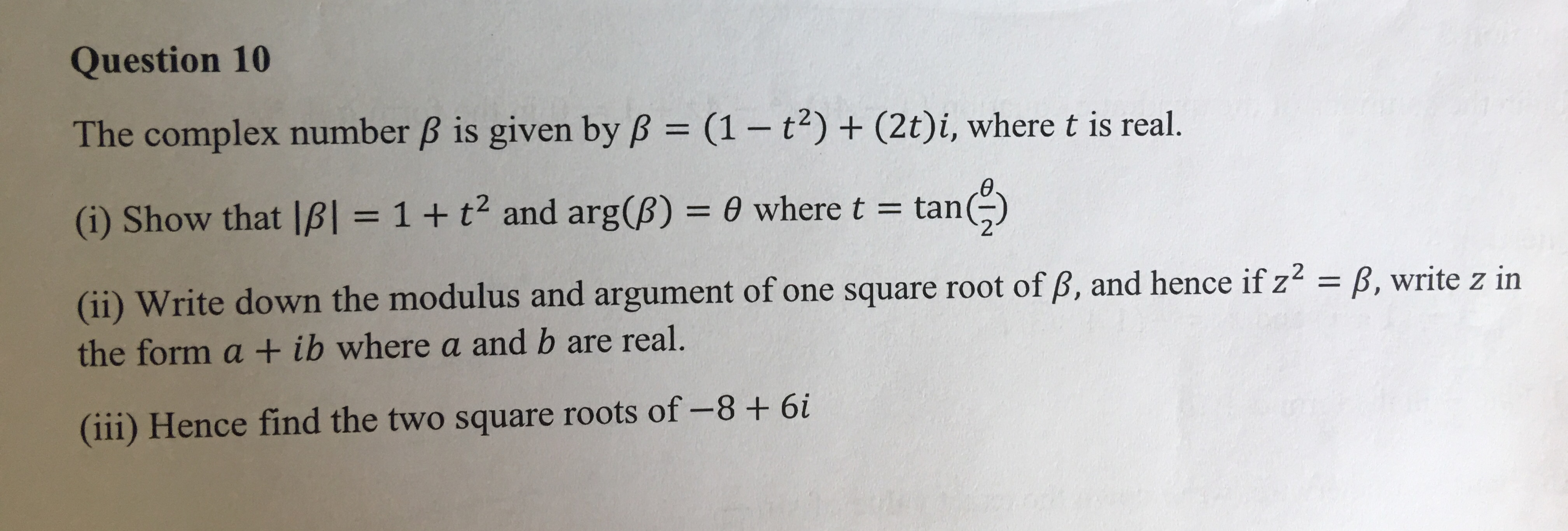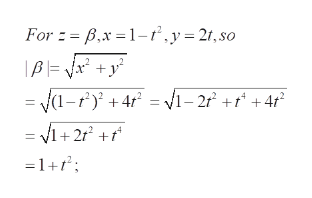# Question 10The complex number B is given by B (1- t) + (2t)i, where t is real.IItan)(i) Show that IB = 1 + t2 and arg(B) 0 where t = t(ii) Write down the modulus and argument of one square root of B, and hence if z2 = B, write z inthe form a + ib where a and b are real.(iii) Hence find the two square roots of -8+ 6i

Question
1 views

[Lin WS - Question 10]

Hi, Question is Attachedhelp_outlineImage TranscriptioncloseQuestion 10 The complex number B is given by B (1- t) + (2t)i, where t is real. II tan) (i) Show that IB = 1 + t2 and arg(B) 0 where t = t (ii) Write down the modulus and argument of one square root of B, and hence if z2 = B, write z in the form a + ib where a and b are real. (iii) Hence find the two square roots of -8+ 6i fullscreen
check_circle

Step 1

The problem concerns the algebraic operations in Complex numbers

Step 2

Definitions and notations : here x is the real part and y the imaginary part of the complex number z.

Step 3

Computing |B...help_outlineImage TranscriptioncloseFor z B,x 1-.y- 21, so = Y(1-f + 4r = vi-2r +r +4r = 1+2r2 +f° =1+r fullscreen

### Want to see the full answer?

See Solution

#### Want to see this answer and more?

Solutions are written by subject experts who are available 24/7. Questions are typically answered within 1 hour.*

See Solution
*Response times may vary by subject and question.
Tagged in

### Math